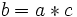# Left-divisor relation in a semigroup

## Definition

Let$(S,*)$ be a semigroup (a set$S$ with associative binary operation$*$). The left-divisor relation in$S$ is defined as follows. For$a,b \in S$, we say that$a$ is a left divisor of$b$ if there exists$c \in S$ such that$b = a * c$.

## Facts

### The relation is transitive

This follows from associativity.

### The relation is reflexive for monoids and idempotent semigroups

If$S$ is a monoid with identity element$e$, then$a$ is a left divisor of$a$ for all$a$, because$a * e = a$.

If$S$ is an idempotent semigroup (a semigroup where every element is idempotent), then$a$ is a left divisor of$a$ for every$a \in S$, because$a * a = a$.

Thus, the relation gives a quasiorder on$S$ when$S$ is a monoid or idempotent semigroup.